Miscellaneous

Chapter 9 Class 11 Straight Lines
Serial order wise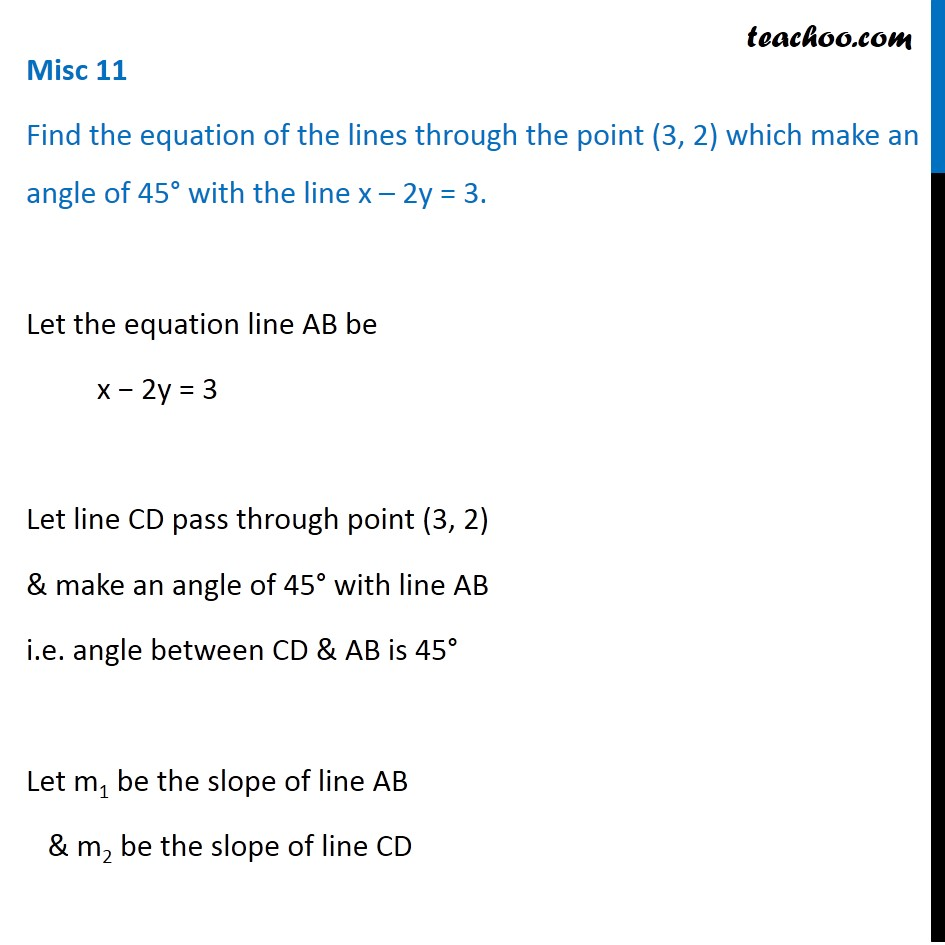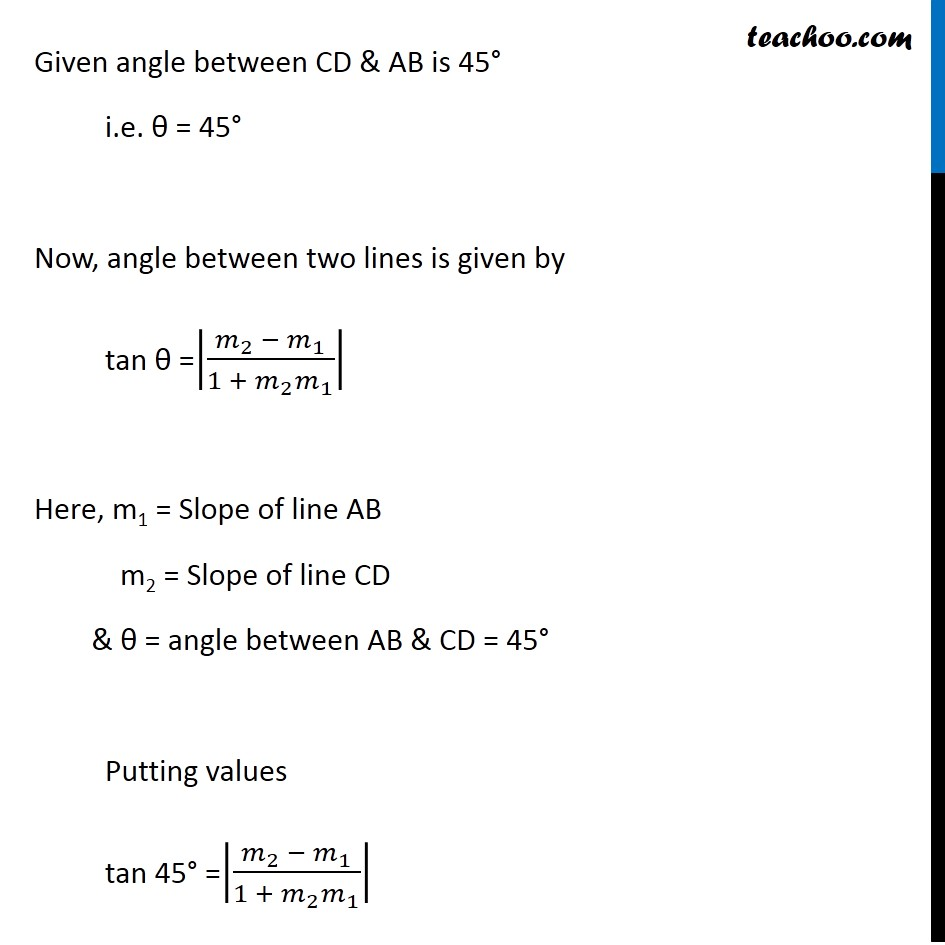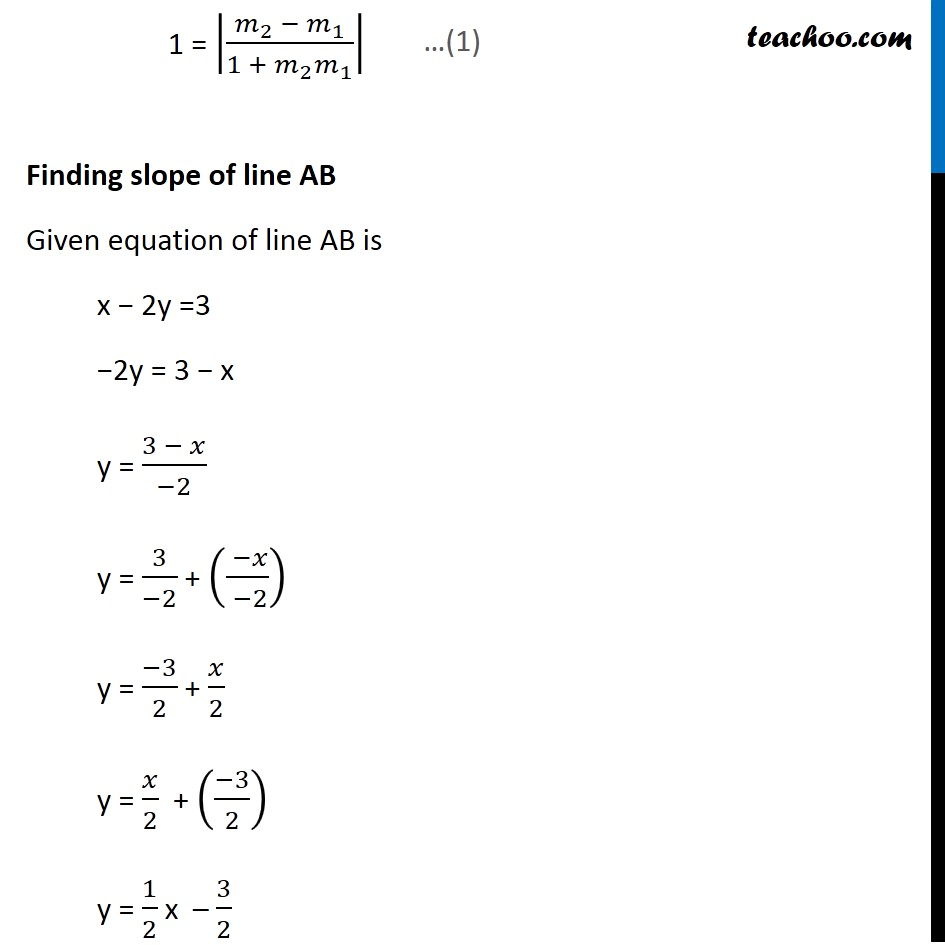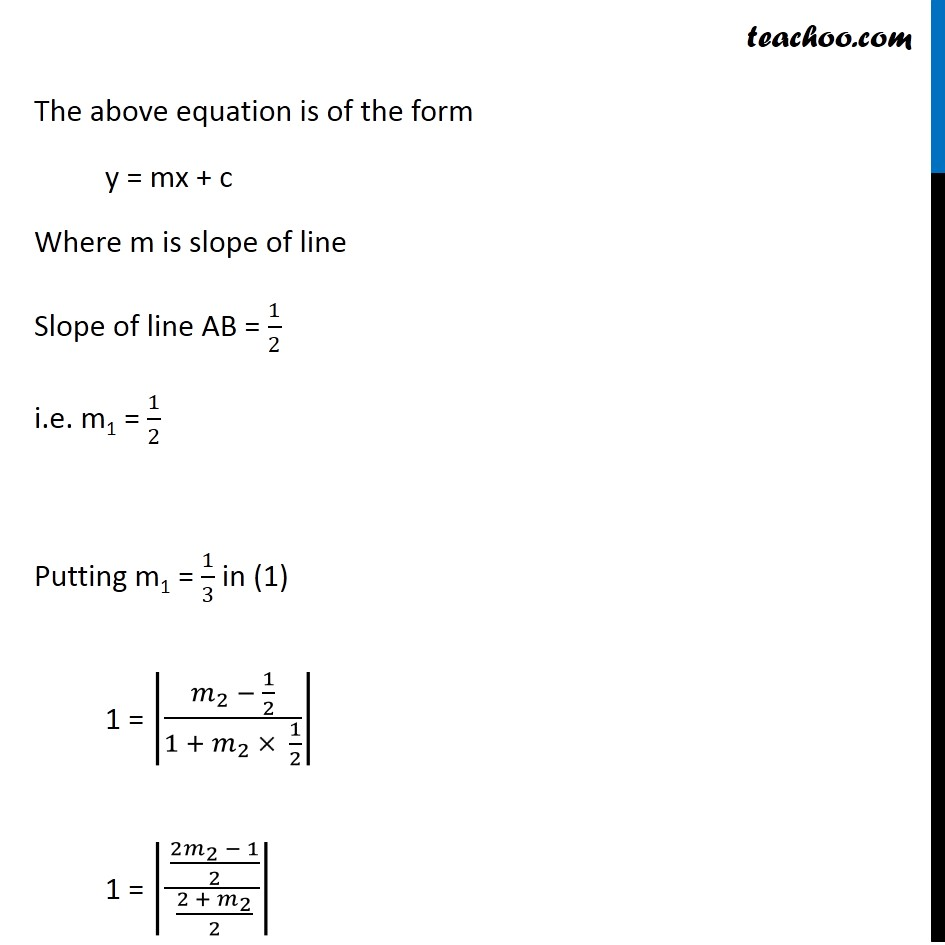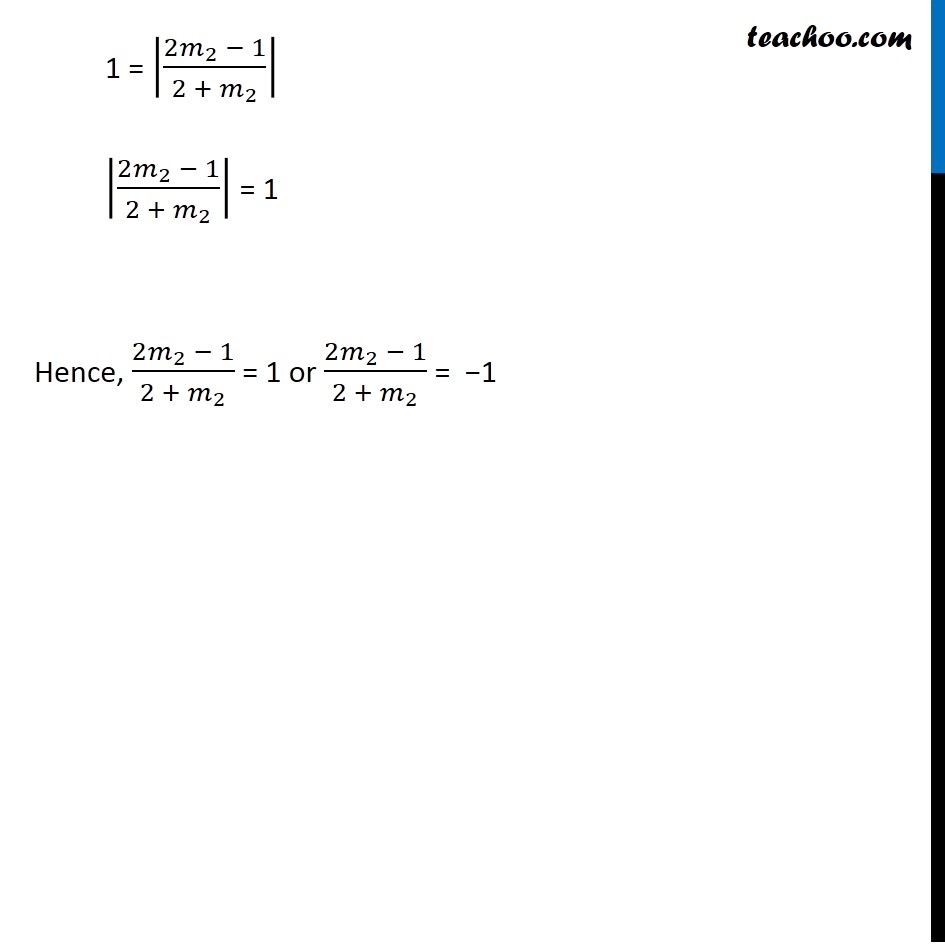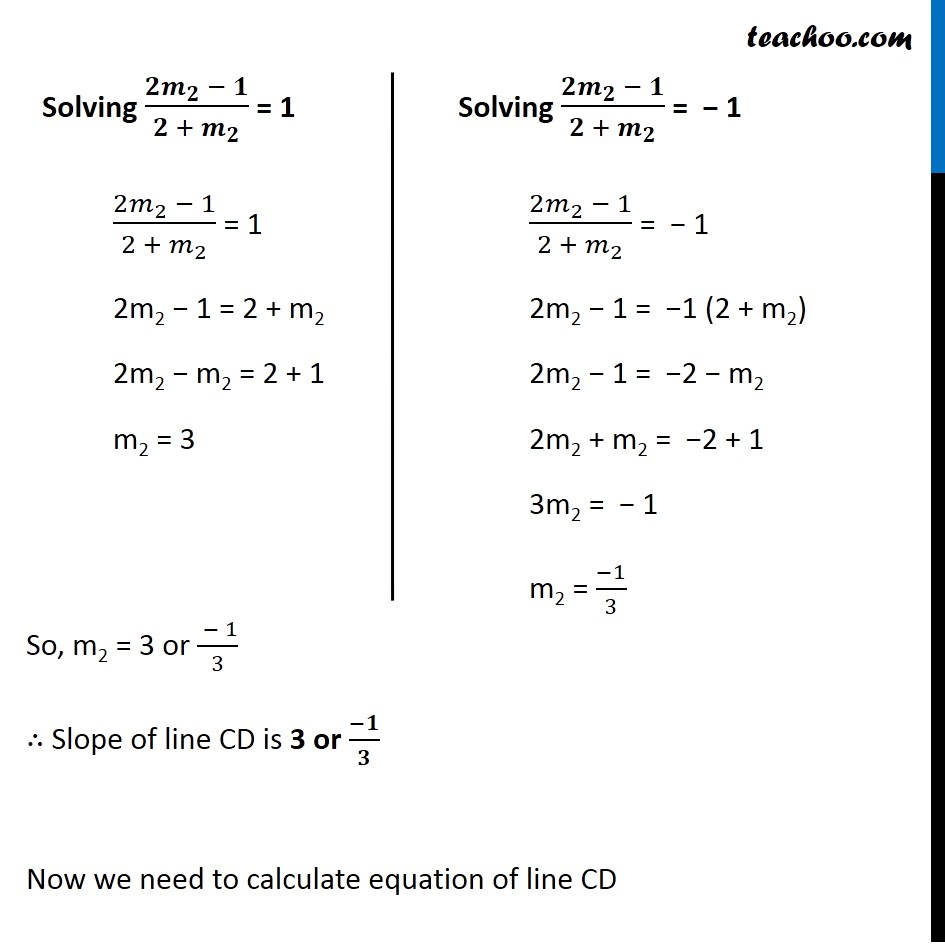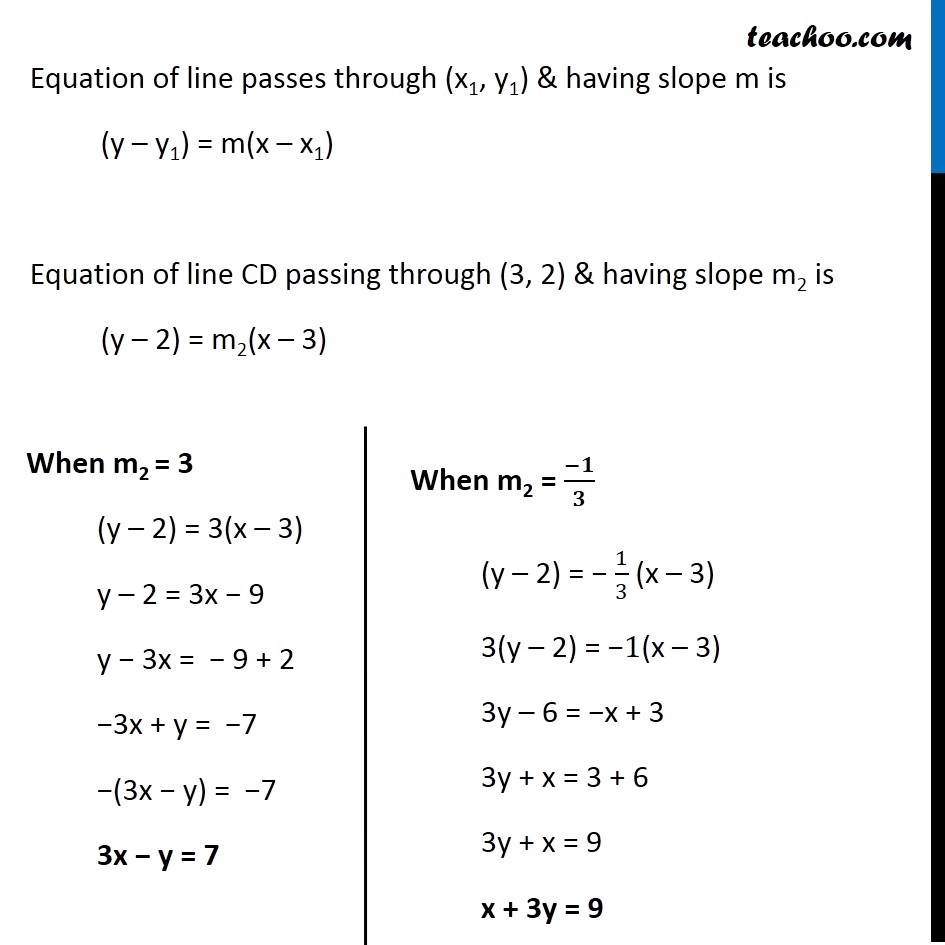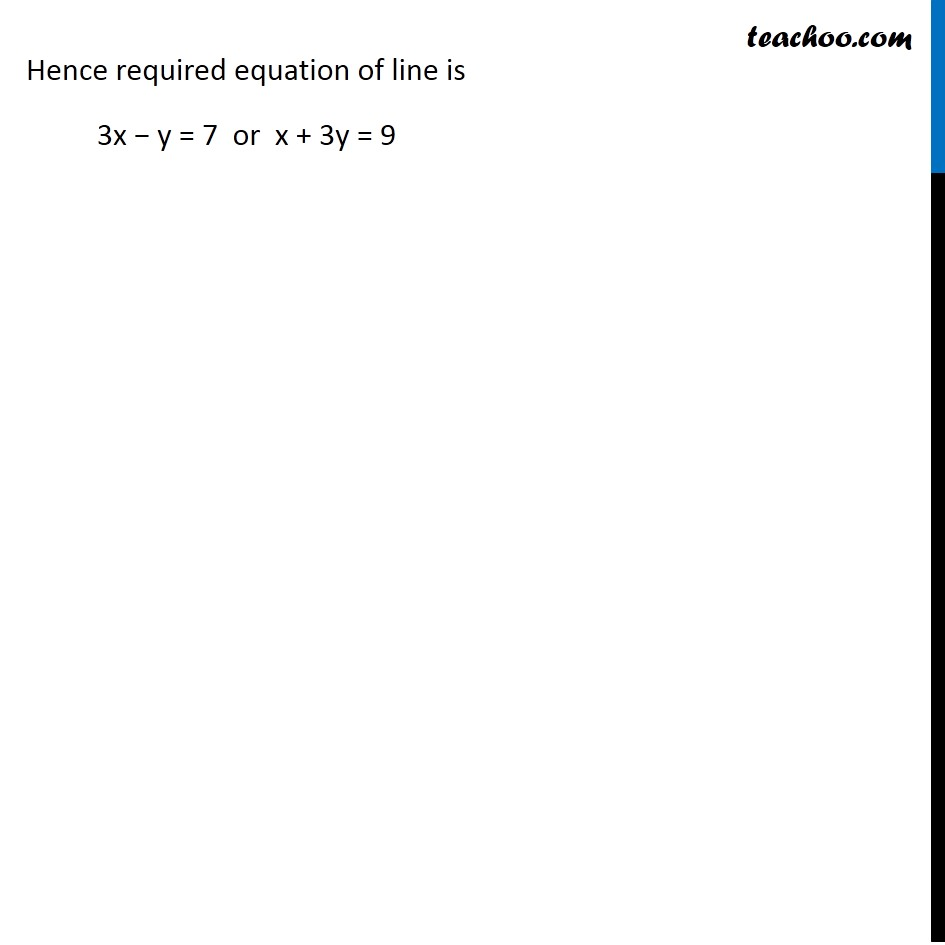Learn in your speed, with individual attention - Teachoo Maths 1-on-1 Class

### Transcript

Misc 10 Find the equation of the lines through the point (3, 2) which make an angle of 45° with the line x – 2y = 3. Let the equation line AB be x − 2y = 3 Let line CD pass through point (3, 2) & make an angle of 45° with line AB i.e. angle between CD & AB is 45° Let m1 be the slope of line AB & m2 be the slope of line CD Given angle between CD & AB is 45° i.e. θ = 45° Now, angle between two lines is given by tan θ =|(𝑚_2 − 𝑚_1)/(1 + 𝑚_2 𝑚_1 )| Here, m1 = Slope of line AB m2 = Slope of line CD & θ = angle between AB & CD = 45° Putting values tan 45° =|(𝑚_2 − 𝑚_1)/(1 + 𝑚_2 𝑚_1 )| 1 = |(𝑚_2 − 𝑚_1)/(1 + 𝑚_2 𝑚_1 )| Finding slope of line AB Given equation of line AB is x − 2y =3 −2y = 3 − x y = (3 − 𝑥)/(−2) y = 3/(−2) + (( −𝑥)/( −2)) y = (−3)/2 + 𝑥/2 y = 𝑥/2 + ((−3)/2) y = 1/2 x – 3/2 The above equation is of the form y = mx + c Where m is slope of line Slope of line AB = 1/2 i.e. m1 = 1/2 Putting m1 = 1/3 in (1) 1 = |(𝑚_2 − 1/2)/(1 + 𝑚_2 × 1/2)| 1 = |( (2𝑚_2 − 1)/2)/( (2 + 𝑚_2)/2)| 1 = |(2𝑚_2 − 1)/(2 + 𝑚_2 )| |(2𝑚_2 − 1)/(2 + 𝑚_2 )| = 1 Hence, (2𝑚_2 − 1)/(2 + 𝑚_2 ) = 1 or (2𝑚_2 − 1)/(2 + 𝑚_2 ) = −1 Solving (𝟐𝒎_𝟐 − 𝟏)/(𝟐 + 𝒎_𝟐 ) = 1 (2𝑚_2 − 1)/(2 + 𝑚_2 ) = 1 2m2 − 1 = 2 + m2 2m2 − m2 = 2 + 1 m2 = 3 Solving (𝟐𝒎_𝟐 − 𝟏)/(𝟐 + 𝒎_𝟐 ) = − 1 (2𝑚_2 − 1)/(2 + 𝑚_2 ) = − 1 2m2 − 1 = −1 (2 + m2) 2m2 − 1 = −2 − m2 2m2 + m2 = −2 + 1 3m2 = − 1 m2 = (−1)/3 So, m2 = 3 or ( − 1)/3 ∴ Slope of line CD is 3 or (−𝟏)/𝟑 Now we need to calculate equation of line CD Equation of line passes through (x1, y1) & having slope m is (y – y1) = m(x – x1) Equation of line CD passing through (3, 2) & having slope m2 is (y – 2) = m2(x – 3) When m2 = 3 (y – 2) = 3(x – 3) y – 2 = 3x − 9 y − 3x = − 9 + 2 −3x + y = −7 −(3x − y) = −7 3x − y = 7 When m2 = (−𝟏)/𝟑 (y – 2) = − 1/3 (x – 3) 3(y – 2) = −1(x – 3) 3y – 6 = −x + 3 3y + x = 3 + 6 3y + x = 9 x + 3y = 9 Hence required equation of line is 3x − y = 7 or x + 3y = 9